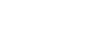xMass concentration (chemistry)Encyclopedia
In chemistry
Chemistry
Chemistry is the science of matter, especially its chemical reactions, but also its composition, structure and properties. Chemistry is concerned with atoms and their interactions with other atoms, and particularly with the properties of chemical bonds....

, the mass concentration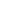(or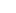) is defined as the mass
Mass
Mass can be defined as a quantitive measure of the resistance an object has to change in its velocity.In physics, mass commonly refers to any of the following three properties of matter, which have been shown experimentally to be equivalent:...

of a constituent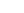divided by the volume
Volume
Volume is the quantity of three-dimensional space enclosed by some closed boundary, for example, the space that a substance or shape occupies or contains....

of the mixture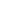: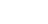## Definition and properties

The volumein the definition refers to the volume of the solution, not the volume of the solvent
Solvent
A solvent is a liquid, solid, or gas that dissolves another solid, liquid, or gaseous solute, resulting in a solution that is soluble in a certain volume of solvent at a specified temperature...

. One liter of a solution usually contains either slightly more or slightly less than 1 liter of solvent because the process of dissolution causes volume of liquid to increase or decrease.

### Dependence on volume

Mass concentration depends on the variation of the volume of the solution due mainly to thermal expansion.

### Sum of mass concentration

The sum of the mass concentrations of all components (including the solvent) gives the density
Density
The mass density or density of a material is defined as its mass per unit volume. The symbol most often used for density is ρ . In some cases , density is also defined as its weight per unit volume; although, this quantity is more properly called specific weight...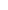of the solution: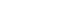Thus, for pure component the mass concentration equals the density of the component.

## Units

The SI-unit
International System of Units
The International System of Units is the modern form of the metric system and is generally a system of units of measurement devised around seven base units and the convenience of the number ten. The older metric system included several groups of units...

for mass concentration is kg/m3 (kilogram
Kilogram
The kilogram or kilogramme , also known as the kilo, is the base unit of mass in the International System of Units and is defined as being equal to the mass of the International Prototype Kilogram , which is almost exactly equal to the mass of one liter of water...

/cubic metre
Cubic metre
The cubic metre is the SI derived unit of volume. It is the volume of a cube with edges one metre in length. An alternative name, which allowed a different usage with metric prefixes, was the stère...

). However, more commonly the unit g/100mL is used, which is identical to g/dL (gram
Gram
The gram is a metric system unit of mass....

/decilitre).

### Usage in biology

In biology
Biology
Biology is a natural science concerned with the study of life and living organisms, including their structure, function, growth, origin, evolution, distribution, and taxonomy. Biology is a vast subject containing many subdivisions, topics, and disciplines...

, the unit "%" is sometimes incorrectly used to denote mass concentration, also called "weight/volume percentage", or "mass/volume percentage." A solution with 1 g of solute dissolved in a final volume of 100 mL of solution
Solution
In chemistry, a solution is a homogeneous mixture composed of only one phase. In such a mixture, a solute is dissolved in another substance, known as a solvent. The solvent does the dissolving.- Types of solutions :...

would be labeled as "1 %" or "1 % w/v" (weight/volume). The notation is mathematically flawed because the unit "%" can only be used for dimensionless quantities. "Percent solution" or "percentage solution" are thus terms best reserved for "weight percent solutions" (w/w = wt% = weight solute/weight total solution after mixing), or "volume percent solutions" (v/v = v% = volume solute per volume of total solution after mixing). The very ambiguous terms "percent solution" and "percentage solutions" with no other qualifiers, continue to occasionally be encountered.

This common usage of % to mean wt/v in biology is because of many biological solutions being dilute and water-based or an aqueous solution
Aqueous solution
An aqueous solution is a solution in which the solvent is water. It is usually shown in chemical equations by appending aq to the relevant formula, such as NaCl. The word aqueous means pertaining to, related to, similar to, or dissolved in water...

. Liquid water has a density of approximately 1 g/cm3 (1 g/ml) (water density). Thus 100 ml of water is equal to approximately 100 g. Therefore, a solution with 1 g of solute dissolved in final volume of 100 ml aqueous solution may also be considered 1 % w/w (1 g solute in 100 g water). This approximation breaks down as the solute concentration is increased. For an example, refer to the densities of water-NaCl mixtures (density of water with dissolved NaCl). High solute concentrations are often not physiologically relevant, but are occasionally encountered in pharmacology, where the weight per volume notation is still sometimes encountered. An extreme example is saturated solution of potassium iodide
Potassium iodide
Potassium iodide is an inorganic compound with the chemical formula KI. This white salt is the most commercially significant iodide compound, with approximately 37,000 tons produced in 1985. It is less hygroscopic than sodium iodide, making it easier to work with...

(SSKI) which attains 100 "%" wt/v potassium iodide mass concentration (1 gram KI per mL solution) only because the solubility of the dense salt KI is extremely high in water, and the resulting solution is very dense (1.72 times as dense as water).

Although there are examples to the contrary, it should be stressed that the commonly used "units" of % w/v are grams/milliliters (g/ml). 1% w/v solutions are sometimes thought of as being gram/100 ml but this detracts from the fact that % w/v is g/ml; 1 g of water has a volume of approximately 1 ml (at STP) and the mass concentration is said to be 100%. To make 10 ml of an aqueous 1% cholate solution, 0.1 grams of cholate are dissolved in 10 ml of water. Volumetric flasks are the most appropriate piece of glassware for this procedure as deviations from ideal solution behavior can occur with high solute concentrations.

In solutions, mass concentration is commonly encountered as the ratio of weight/[volume solution], or wt/volume. In water solutions containing relatively small quantities of dissolved solute (as in biology), such figures may be "percentivized" by multiplying by 100 a ratio of grams solute per mL solution. The result is given as "weight/volume percentage," or "mass/volume percentage." Such a convention expresses mass concentration of 1 gram of solute in 100 mL of solution, as "1 w/v %."

### Molar concentration

The conversion to molar concentration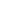is given by: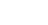where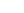is the molar mass
Molar mass
Molar mass, symbol M, is a physical property of a given substance , namely its mass per amount of substance. The base SI unit for mass is the kilogram and that for amount of substance is the mole. Thus, the derived unit for molar mass is kg/mol...

of constituent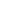.

### Mass fraction

The conversion to mass fraction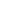is given by: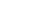### Mole fraction

The conversion to mole fraction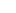is given by: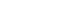where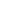is the average molar mass of the mixture.

### Molality

For binary mixtures, the conversion to molalityis given by: